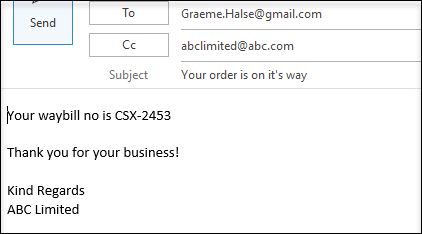# Send Email From Excel With Formula (No Macros!)

This tutorial demonstrates how to send an email from Excel with a formula (no macros necessary).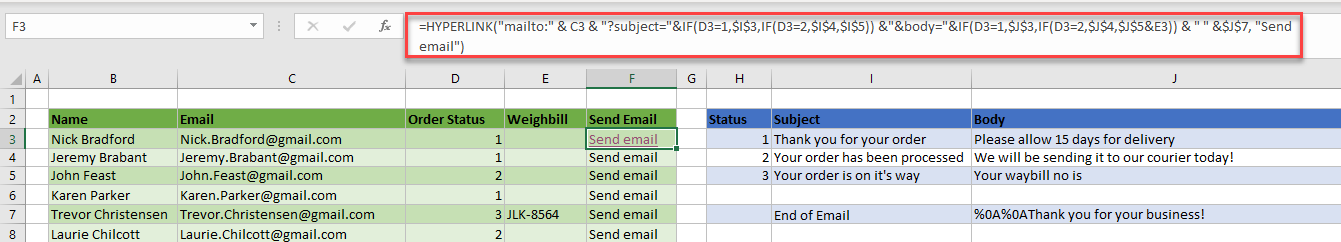In Excel, you can send an email by running a macro, but if you prefer not to use VBA, keep reading.

In Excel, it’s possible to click on a cell and send an email automatically. This can be done with a formula using the HYPERLINK Function. You can create a hyperlink formula that uses the mailto command and auto-populates fields like to, subject, cc, and the body of an email.

``=HYPERLINK("mailto:" & C3 & "?subject="&IF(D3=1,\$I\$3,IF(D3=2,\$I\$4,\$I\$5)) &"&cc=abclimited@abc.com"&"&body="&IF(D3=1,\$J\$3,IF(D3=2,\$J\$4,\$J\$5&E3)) & " " &\$J\$7, "Send email")``

The Link_Location is the first part of the formula. This example sets mailto, subject, and body.

#### Mail To

The “mailto:” part of the formula sets the email recipient. This needs to be a valid email address entered into the formula – or, in this example – linked to a cell (C3) that contains a valid email address.

``"mailto:" & C4``

#### Subject

The “?subject=” can have text between the equals sign and quotation marks, link to a cell, or contain a formula. In this example, there’s a formula to check the status of an order. If the status is 1, use “Thank you for your order” from cell I3.

To do that, use the following IF statement for the subject part of the formula.

``"?subject="&IF(D4=1,\$I\$3,IF(D4=2,\$I\$4,\$I\$5))``

Note that there has to be a ? symbol before the word “subject.”

#### CC

If you want to add a cc to your email, insert it into the formula after the subject in quotation marks. You need an ampersand (&) before the “cc.”. You can either type in the email address to cc the mailto, or you can link it to an external cell as with the subject and body of the email.

``"&cc=abclimited@abc.com"``

#### Body

The “&body=” part of the formula can contain additional text before the closing quotation mark. You can also create a customized IF statement with a formula:

``"&body="&IF(D5=1,\$J\$3,IF(D5=2,\$J\$4,\$J\$5&E5)) & " " &\$J\$7``

This IF statement looks at the status of the order, and then adds a message to the end of the email body (Thank you for your business!).

As with the cc, you need an ampersand (&) before the word “body.”

The message at the end (in cell J7) contains the text:

``"%0A%0AThank you for your business! %0A %0A Kind Regards%0A ABC Limited"``

where the “%0A” adds blank lines. The text in the email is displayed as follows: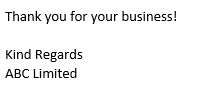Note: In the formula, the cells that refer to the lookup columns (for the subject and body text) have been put in the formulas as absolute (or “locked”) cell addresses. This lets you copy the formula down to the rest of the order records.

### Friendly Name

The final part of the formula is what displays in the hyperlinked cell in Excel – this is the text you click on to create the email. This example uses the text “Send email,” but any text can be entered, as long as it is within quotation marks.

View the formula in the Function Dialog box by clicking on the formula, and then clicking the Insert Function box in the formula bar.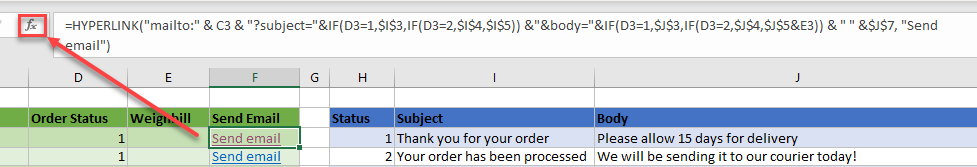The Link_location and Friendly_name are shown in the function arguments.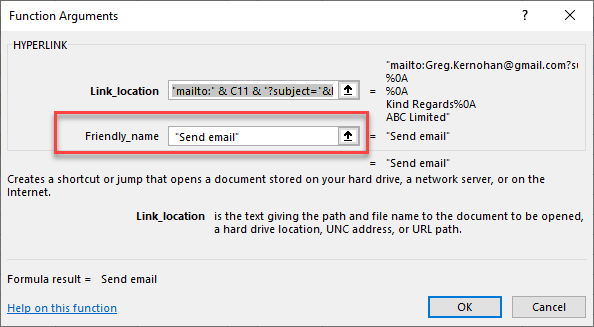Therefore, the formula:

``=HYPERLINK("mailto:" & C3 & "?subject="&IF(D3=1,\$I\$3,IF(D3=2,\$I\$4,\$I\$5)) &"&cc=abclimited@abc.com"&"&body="&IF(D3=1,\$J\$3,IF(D3=2,\$J\$4,\$J\$5&E3)) & " " &\$J\$7, "Send email")``

produces this email: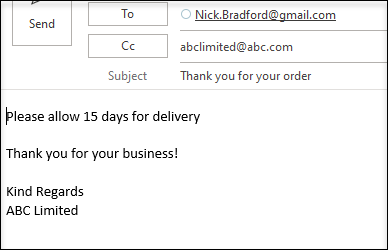## Copy Formula Down

Since the formula uses absolute cell addresses to refer to the subject and body “lookup” cells, you only need to type in the whole formula once. Then, copy the first cell down to the rest of the rows of your range.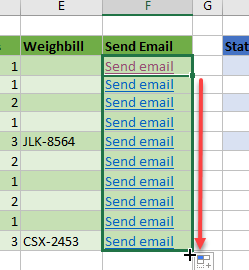Click any of the “Send email” hyperlinks to send a customized email to the client.